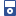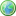Accessibility options:

# Functions and Graphs resources

Show me all resources applicable to

### iPOD Video (7)Introduction to Functions - Part 1
IPOD VIDEO: A function is a rule which operates on one number to give another number. However, not every rule describes a valid function. This unit explains how to see whether a given rule describes a valid function, and introduces some of the mathematical terms associated with functions. This resource is released under a Creative Commons license Attribution-Non-Commercial-No Derivative Works and the copyright is held by Skillbank Solutions Ltd.Introduction to Functions - Part 2
IPOD VIDEO: A function is a rule which operates on one number to give another number. However, not every rule describes a valid function. This unit explains how to see whether a given rule describes a valid function, and introduces some of the mathematical terms associated with functions. This resource is released under a Creative Commons license Attribution-Non-Commercial-No Derivative Works and the copyright is held by Skillbank Solutions Ltd.Introduction to Functions - Part 3
IPOD VIDEO: A function is a rule which operates on one number to give another number. However, not every rule describes a valid function. This unit explains how to see whether a given rule describes a valid function, and introduces some of the mathematical terms associated with functions. This resource is released under a Creative Commons license Attribution-Non-Commercial-No Derivative Works and the copyright is held by Skillbank Solutions Ltd.Introduction to Functions - Part 4
IPOD VIDEO: A function is a rule which operates on one number to give another number. However, not every rule describes a valid function. This unit explains how to see whether a given rule describes a valid function, and introduces some of the mathematical terms associated with functions. This resource is released under a Creative Commons license Attribution-Non-Commercial-No Derivative Works and the copyright is held by Skillbank Solutions Ltd.Introduction to Functions - Part 5
IPOD VIDEO: A function is a rule which operates on one number to give another number. However, not every rule describes a valid function. This unit explains how to see whether a given rule describes a valid function, and introduces some of the mathematical terms associated with functions. This resource is released under a Creative Commons license Attribution-Non-Commercial-No Derivative Works and the copyright is held by Skillbank Solutions Ltd.Introduction to Functions - Part 6
IPOD VIDEO: A function is a rule which operates on one number to give another number. However, not every rule describes a valid function. This unit explains how to see whether a given rule describes a valid function, and introduces some of the mathematical terms associated with functions. This resource is released under a Creative Commons license Attribution-Non-Commercial-No Derivative Works and the copyright is held by Skillbank Solutions Ltd.Limits of functions
Video for iPod. This resource is released under a Creative Commons license Attribution-Non-Commercial-No Derivative Works and the copyright is held by Skillbank Solutions Ltd.

### Quick Reference (7)Graphs of the trigonometric functions
This leaflet gives graphs of the functions sine, cosine and tangent and notes some important properties. (Engineering Maths First Aid Kit 4.3)The exponential constant e
This leaflet gives some basic information about the constant e. It shows graphs of the exponential function. (Engineering Maths First Aid Kit 3.4)The graph of a function
This leaflet reminds students of important conventions associated with graph plotting. It explains the terms dependent variable and independent variable.(Engineering Maths First Aid Kit 3.2)The hyperbolic functions
This leaflet gives definitions of the hyperbolic functions in terms of exponential functions. Graphs of the hyperbolic sine, cosine and tangent are illustrated. (Engineering Maths First Aid Kit 3.5)The hyperbolic identities
This leaflet lists common identities involving the hyperbolic functions. (Engineering Maths First Aid Kit 3.6)The logarithm function
This leaflet provides a table of values and a graph of the logarithm function. (Engineering Maths First Aid Kit 3.7)What is a function?
This introductory leaflet explains what is meant by a function, gives functional notation, and some simple examples. (Engineering Maths First Aid Kit 3.1)

### Teach Yourself (9)Composition of functions
We can build up complicated functions from simple functions by using the process of composition, where the output of one function becomes the input of another. It is also sometimes necessary to carry out the reverse process, decomposing a complicated function into two or more simple functions. This unit explains how.Exponential and logarithm functions
Exponential functions and logarithm functions are important in both theory and practice. In this unit we look at the graphs of exponential and logarithm functions, and see how they are related.Hyperbolic functions
The hyperbolic functions have similar names to the trigonmetric functions, but they are defined in terms of the exponential function. In this unit we define the three main hyperbolic functions, and sketch their graphs. We also discuss some identities relating these functions, and mention their inverse functions and reciprocal functions.Introduction to functions
A function is a rule which operates on one number to give another number. However, not every rule describes a valid function. This unit explains how to see whether a given rule describes a valid function, and introduces some of the mathematical terms associated with functions.Inverse functions
An inverse function is a second function which undoes the work of the first one. In this unit we describe two methods for finding inverse functions, and we also explain that the domain of a function may need to be restricted before an inverse function can exist.Limits of functions
In this unit, we explain what it means for a function to tend to infinity, to minus infinity, or to a real limit, as x tends to infinity or to minus infinity. We also explain what it means for a function to tend to a real limit as x tends to a given real number. In each case, we give an example of a function that does not tend to a limit at all.Linear functions
Some of the most important functions are linear. This unit describes how to recognize a linear function, and how to find the slope and the y-intercept of its graph.Polynomial functions
Many common functions are polynomial functions. In this unit we describe polynomial functions and look at some of their properties.Trigonometric functions
The sine, cosine and tangent of an angle are all defined in terms of trigonometry, but they can also be expressed as functions. In this unit we examine these functions and their graphs. We also see how to restrict the domain of each function in order to define an inverse function.

### Test Yourself (2)Maths EG
Computer-aided assessment of maths, stats and numeracy from GCSE to undergraduate level 2. These resources have been made available under a Creative Common licence by Martin Greenhow and Abdulrahman Kamavi, Brunel University.Trigonometry Test 01 (DEWIS)
Five questions on trigonometry. The first involves determining the quadrant an angle lies in, the remaining questions involve solving trigonometric equations. DEWIS resources have been made available under a Creative Commons licence by Rhys Gwynllyw & Karen Henderson, University of the West of England, Bristol.

### Third Party Resources (1)Mathematics Support Materials from the University of Plymouth
Support material from the University of Plymouth:
The output from this project is a library of portable, interactive, web based support packages to help students learn various mathematical ideas and techniques and to support classroom teaching.
There are support materials on ALGEBRA, GRAPHS, CALCULUS, and much more.
This material is offered through the mathcentre site courtesy of Dr Martin Lavelle and Dr Robin Horan from the University of Plymouth.

### Video (11)Completing the square - an Animation
This mathtutor animation shows how the quadratic equation for a parabola may be transformed by completing the square. This resource is released under a Creative Commons license Attribution-Non-Commercial-No Derivative Works and the copyright is held by Skillbank Solutions Ltd.Composition of functions
We can build up complicated functions from simple functions by using the process of composition, where the output of one function becomes the input of another. It is also sometimes necessary to carry out the reverse process, decomposing a complicated function into two or more simple functions. This unit explains how. (Mathtutor Video Tutorial) This resource is released under a Creative Commons license Attribution-Non-Commercial-No Derivative Works and the copyright is held by Skillbank Solutions Ltd.Exponential and logarithm functions
Exponential functions and logarithm functions are important in both theory and practice. In this unit we look at the graphs of exponential and logarithm functions, and see how they are related. (Mathtutor Video Tutorial) This resource is released under a Creative Commons license Attribution-Non-Commercial-No Derivative Works and the copyright is held by Skillbank Solutions Ltd.Hyperbolic functions
The hyperbolic functions have similar names to the trigonmetric functions, but they are defined in terms of the exponential function. In this unit we define the three main hyperbolic functions, and sketch their graphs. We also discuss some identities relating these functions, and mention their inverse functions and reciprocal functions. (Mathtutor Video Tutorial) This resource is released under a Creative Commons license Attribution-Non-Commercial-No Derivative Works and the copyright is held by Skillbank Solutions Ltd.Hyperbolic functions - David Saunders
This mathtutor extention video explains the derivation of hyperbolic functions starting from two-dimensional space. This resource is released under a Creative Commons license Attribution-Non-Commercial-No Derivative Works and the copyright is held by Skillbank Solutions Ltd.Introduction to functions
A function is a rule which operates on one number to give another number. However, not every rule describes a valid function. This unit explains how to see whether a given rule describes a valid function, and introduces some of the mathematical terms associated with functions. (Mathtutor Video Tutorial) This resource is released under a Creative Commons license Attribution-Non-Commercial-No Derivative Works and the copyright is held by Skillbank Solutions Ltd.Inverse functions
An inverse function is a second function which undoes the work of the first one. In this unit we describe two methods for finding inverse functions, and we also explain that the domain of a function may need to be restricted before an inverse function can exist. (Mathtutor Video Tutorial) This resource is released under a Creative Commons license Attribution-Non-Commercial-No Derivative Works and the copyright is held by Skillbank Solutions Ltd.Limits of functions
In this unit, we explain what it means for a function to tend to infinity, to minus infinity, or to a real limit, as x tends to infinity or to minus infinity. We also explain what it means for a function to tend to a real limit as x tends to a given real number. In each case, we give an example of a function that does not tend to a limit at all. (Mathtutor Video Tutorial) This resource is released under a Creative Commons license Attribution-Non-Commercial-No Derivative Works and the copyright is held by Skillbank Solutions Ltd.Linear functions
Some of the most important functions are linear. This unit describes how to recognize a linear function, and how to find the slope and the y-intercept of its graph. (Mathtutor Video Tutorial) This resource is released under a Creative Commons license Attribution-Non-Commercial-No Derivative Works and the copyright is held by Skillbank Solutions Ltd.Polynomial functions
Many common functions are polynomial functions. In this unit we describe polynomial functions and look at some of their properties. (Mathtutor Video Tutorial) This resource is released under a Creative Commons license Attribution-Non-Commercial-No Derivative Works and the copyright is held by Skillbank Solutions Ltd.Trigonometric functions
The sine, cosine and tangent of an angle are all defined in terms of trigonometry, but they can also be expressed as functions. In this unit we examine these functions and their graphs. We also see how to restrict the domain of each function in order to define an inverse function. (Mathtutor Video Tutorial) This resource is released under a Creative Commons license Attribution-Non-Commercial-No Derivative Works and the copyright is held by Skillbank Solutions Ltd.

Website design by Pink Mayhem, Leicester